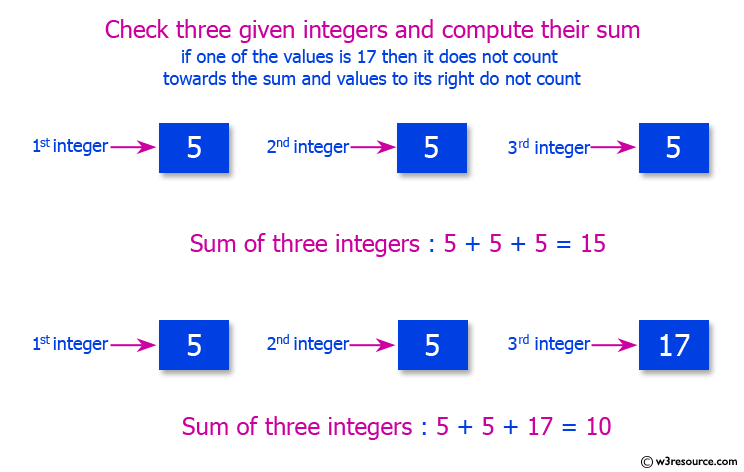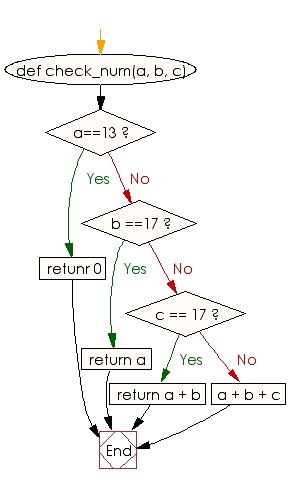﻿ Ruby Basic exercises: Check three given integers and compute their sum - w3resource# Ruby Basic Exercises: Check three given integers and compute their sum

## Ruby Basic: Exercise-53 with Solution

Write a Ruby program to check three given integers and compute their sum. However, if one of the values is 17 then it does not count towards the sum and values to its right do not count.Ruby Code:

``````def check_num(a, b, c)
if a==13
retunr 0
elsif b ==17
return a
elsif c == 17
return a + b
else
a + b + c
end
end
print check_num(5, 5, 5),"\n"
print check_num(5, 5, 17)
``````

Output:

```15
10
```

Flowchart:Ruby Code Editor: